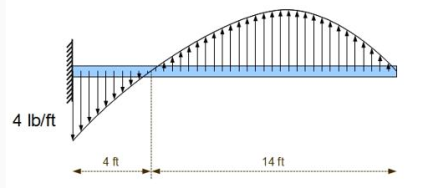## Question:

Find the point load equivalent to the parabolic distributed load shown. The load is parabolic with maximum load equal to 500 lb/ft. The dimensions on the beam are a = 4 ft, b = 4 ft, and c = 2 ft. What are the reactions at the wall?## Vector Quantities & Scalar Quantities:

Vector quantities are those that have not only magnitude (size) but also direction, for example, acceleration, displacement, force, momentum, and velocity. By comparison, scalar quantities are those that are characterized by their magnitude only.

Become a Study.com member to unlock this answer!

Given Data

• The given parallel vector is {eq}\overrightarrow a = i - 2j {/eq}.
• The magnitude of the vector is {eq}\left| b \right| = 10 {/eq}.

...Parabolic Path: Definition & Projectiles

from

Chapter 14 / Lesson 13
27K

Parabolic path is defined as the angle of trajectory of a projectile. Learn the definition of parabolic path, projectiles, initial velocity, and see examples of how to calculate the parabolic path of a projectile.